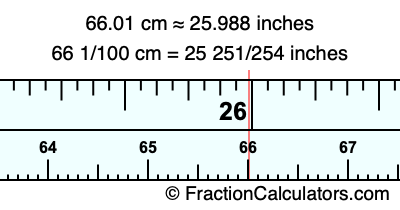66.01 cm in inchesHere is how to convert 66.01 cm to inches. We will give you the fractional answer, the decimal answer, and illustrate the answer on our tape measure.

One centimeter is equal to 50/127 inches, and 66.01 as a fraction is 66 1/100. Therefore, to get the fractional answer to 66.01 cm in inches, we multiply 66 1/100 by 50/127 and simplify if necessary.

cm × 50/127 = inches
66 1/100 × 50/127 = inches
66 1/100 × 50/127 = 25 251/254
66 1/100 cm = 25 251/254 inches
66.01 cm = 25 251/254 inches

As promised above, we also have the answer to 66.01 cm in inches in decimal form. Although the fractional answer above is exact, the decimal answer may be rounded if necessary:

25 251/254 ≈ 25.988188976378
25 251/254 ≈ 25.988
66.01 cm ≈ 25.988 inches

Our image below shows 66.01 cm on a tape measure. The top row of the tape measure is inches, and the bottom row is centimeters.As you can see, we drew a red line where 66.01 cm and 25.988 inches meet on the measuring tape.

Centimeters to Inches Calculator
Here you can convert another length in centimeters to inches.

Convert  cm to inches.

What is 66.02 cm in inches?
Here is another inches to centimeters calculation we did for you.